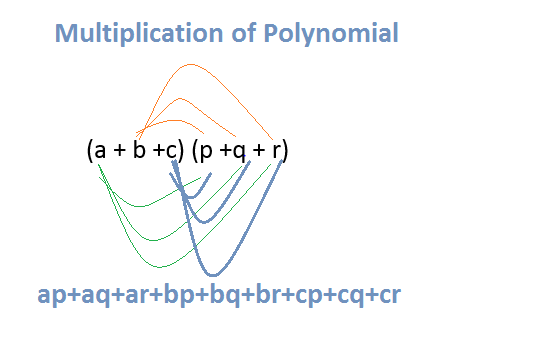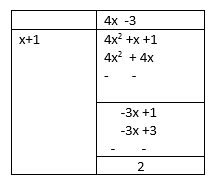# Multiplying Polynomials|Dividing Polynomials

Table of Content

Welcome to Class 9 Maths chapter 2 Polynomials notes.The topics in this page are Multiplying Polynomials and Dividing Polynomials along with examples, and questions and answers .This is according to CBSE and the NCERT textbook. If you like the study material, feel free to share the link as much as possible.

## Multiplying polynomials

Multiplication of polynomial is simple task and we can follow below steps to multiply polynomials

1. Arrange both the polynomial is same order of exponent . It would be good to have terms arrange from highest exponent to lowest exponent
2. We have to distribute each term of the first polynomial to every term of the second polynomial.
a. when you multiply two terms together you must multiply the coefficient (numbers) and add the exponents
b. Also as we already know ++ equals =, +- or -+ equals - and -- equals +
3. group like termsSo multiplication may involve
• Multiplication of monomial to monomial
• Multiplication of monomial to binomial, trinomial or more terms polynomials
• Multiplication of binomial, trinomial or more terms polynomials to monomial
• Multiplication of binomial to binomial, trinomial or more terms polynomials
• Multiplication of trinomial to trinomial or more terms polynomials
Multiply the Monomials
1. $x^2 \times (2x^{22}) \times (4x^26)$
2. $2 (\frac {-10xy^3}{3}) \times (\frac {6x^3y}{5})$
3. $( x) \times (x^2) \times (x^3) \times (x^8)$
We will use the below property extensively in above questions
$x^m \times x^n \times x^o=x^{m+n+o}$
1. As you know from above
So, we get
$x^2 \times (2x^{22}) \times (4x^{26}) =8a^{48}$
2. $2 (\frac {-10xy^3}{3}) \times (\frac {6x^3y}{5})$ $=(-8x^4y^4)$
3.$( x) \times (x^2) \times (x^3) \times (x^8)$
$=x^{14}$
Multiply the binomials.
(i) (2x + 5) and (4x - 3)
(ii) (x - 8) and (3x - 4)
(iii)(2.5x - 0.5) and (2.5x + 0.5)
Let  $( a+b) (c+d)$ to be done
then
$( a+b) (c+d)= a(c+d) + b( c+d)$
$=(a \times c)+(a \times d)+(b \times c)+(b \times d)$
The above expression of multiplying binomials is called FOIL method
FOIL stands FIRST ($a \times c$) OUTER ($a \times d$) INNER ($b \times c$) LAST($b \times d$)
We will use the same concept in all the question below
1. $(2x + 5)(4x - 3)$
$=2x \times 4x - 2x \times 3 +5 \times 4x- 5 \times 3$
$=8x^2 - 6x + 20x -15$
$=8x^2 + 14x -15$
2. $( y - 8)(3y - 4)$
$= y \times 3y - 4y - 8 \times 3y + 32$
$= 3y^2- 4y - 24y + 32$
$= 3y^2- 28y + 32$
3. $(2.5l - 0.5m)(2.5l + 0.5)$
Using $(a+b)(a-b) = a^2- b^2$
We get ,
$= 6.25l^2- 0.25m^2$
Multiply Binomial by Trinomial
$(3x + 2)(4x^2 - 7x + 5)$
Let  $( a+b) (c+d+e)$ to be done
then
$( a+b) (c+d+e)= a(c+d+e) + b( c+d+e)$
$=(a \times c)+(a \times d)+ (a \times e)+ (b \times c)+(b \times d) +(b \times e)$
We will use the same concept in all the question below
$=3x(4x^2 - 7x + 5) + 2(4x^2 - 7x + 5)$
$=12x^3 -21x^2 +15x +8x^2 -14x+10$
$=12x^3 -13x^2 +x+10$

Practice Questions
• $(x-5)(x-9)$
• $\left(x + 10\right) \left(x^{2} + x + 2\right)$
• $3x^2 \times 4x^{20}$
• $\left(x + 4\right) \left(x^{2} + 4 x + 7\right)$
• $(x+9)(x-6)$
• $\left(x + 2\right) \left(x + 4\right)^{2}$
• $\left(x + 8\right) \left(x^{2} + 2 x + 9\right)$
• $(x-2)(x-2)$

## Dividing Polynomials

When a polynomial p(x) is divided by the polynomial g(x), we get quotient q(x) and remainder r(x)

$p(x)=g(x).q(x)+r(x)$
Notes
1. The degree of the reminder r(x) is always less then divisor g(x)

Now Let us see how to divide the polynomial by another non-zero polynomial

Steps to divide a polynomial by another polynomial. This is also called the long division method of polynomials

1. Arrange the term in decreasing order in both the polynomial
2. Divide the highest degree term of the dividend by the highest degree term of the divisor to obtain the first term
3. Now We multiply the divisor by the first term of the quotient, and subtract this product from the dividend
4. Similar steps are followed till we get the reminder whose degree is less than of divisor
Example
Divide p(x) by g(x), where $p(x) = x + 4x^2 -1$ and $g(x) = 1 + x$
Solution
We carry out the process of division by means of the following steps:
Step 1 We write the dividend $x + 4x^2 - 1$ and the divisor 1 + x. in the standard form, i.e., after arranging the terms in the descending order of their degrees. So, the dividend is $4x^2 + x -1$ and divisor is x + 1.
Step 2We divide the first term of the dividend by the first term of the divisor, i.e., we divide $4x^2$ by x, and get 4x. This gives us the first term of the quotient.
Step 3We multiply the divisor by the first term of the quotient, and subtract this product from the dividend, i.e., we multiply $x + 1$ by 4x and subtract the product $4x^2 + 4x$ from the dividend $4x^2 + x - 1$. This gives us the remainder as -3x - 1.
Step 4 We treat the remainder -3x - 1 as the new dividend. The divisor remains the same. We repeat Step 2 to get the next term of the quotient, i.e., we divide the first term -3x of the (new) dividend by the first term x of the divisor and obtain -3. Thus, -3 is the second term in the quotient.
Step 5 We multiply the divisor by the second term of the quotient and subtract the product from the dividend. That is, we multiply x + 1 by - 3 and subtract the product -3x - 3 from the dividend - 3x - 1. This gives us 2 as the remainder

Now the degree of the reminder is less than degree of the divisor so process stops here

Complete division is illustrated belowQuotient is 3x -3 and Reminder is 2
Now $4x^2 + x -1= (x+1)(4x-3) +2$
Practice Questions
• Divide $x^{3} + 12 x^{2} + 39 x + 29$ by $(x+1)$
• Divide $x^{3} + 14 x^{2} + 43 x + 32$ by $(x+3)$
• Divide $x^{3} + 5 x^{2} + 6 x + 2$ by $(x+1)$
• Divide $x^{2} + 2 x - 48$ by $(x+8)$
• Divide $x^{3} + 12 x^{2} + 47 x + 60$ by $(x+3)$

## Remainder Theorem's

If p(x) is an polynomial of degree greater than or equal to 1 and p(x) is divided by the expression (x-a),then the reminder will be p(a)

Important notes
1. for (x-a) then remainder P(a)
2. for (x+a) => x -(-a),then remainder will be P(-a)
3. for (ax-b) => a(x- b/a) ,the remainder will be P(b/a)
4. for (ax+b) => a(x+b/a),the remainder will be P(-b/a)
5. for (b-ax)=> -a(x-b/a),the remainder will be P(b/a)

## Worksheet

### Quiz Time

Question 1 $(x+2)(x+8)$ ?
A. $x^2 +16$
B. $x^2 +16+10x$
C. $x^2 +16+2x$
D. $x^2 +16+8x$
Question 2 $x^2 \times x^3 \times x^{11}$ ?
A. $x^{16}$
B. $x^{17}$
C. $x^{66}$
D. $66x$
Question 3 when $x^{2} - 5 x - 14$ divide by $(x+2)$
A. Remainder is (x-7)
B. Quotient is (x+7)
C. Remainder is 0
D. None of these
Question 4 $\left(x + 9\right) \left(x^{2} + 4 x + 5\right)$
A. $x^{3} + 13 x^{2} - 41 x + 45$
B. $x^{3} + 12 x^{2} + 41 x + 45$
C. $x^{3} + 13 x^{2} + 41 x - 45$
D. $x^{3} + 13 x^{2} + 41 x + 45$
Question 5 $\left(x + 1\right) \left(x + 4\right)^{2}$
A. $x^{3} - 9 x^{2} + 24 x + 16$
B. $x^{3} + 9 x^{2} + 24 x - 16$
C. $x^{3} + 9 x^{2} + 24 x + 16$
D. none of the above
Question 6 $x(x^5+11)$ ?
A. $x^6+11x$
B. $x^6+11$
C. $x^5+11x$
D. none of the above